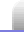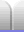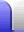#### Volume explained

Volume is measured in cubic metres (m3) or variations on it using prefixes.

The litre (L) is often used to measure volume. A litre is simply a special name for a cubic decimetre.

1 L = 1 dm3

Hence 1 L ≡ 0.001 m3 (exactly). A cubic centimeter, abbreviated as cc, is used to describe the size of car engines. A cc is exactly the same thing as a mL.

1 mL = 1 cm3 = 1 cc

At room temperature at sea level one litre weighs 1 kg. So at room temperature at sea level:

1 mL = 1 cm3 = 1 cc = 1 g
1 L = 1 dm3 = 1 kg
1,000 L = 1 m3 = 1,000 kg (1 tonne)

Note that the symbol for a litre is a capital L, although a lower case l is sometimes used.

### Calculating Volume

#### Volume of a cube:

length x width x height

#### Volume of a prism:

Work out which dimension the prism has been extruded through, then:

area of face x length

4/3·π·r3

### Random facts – heart and river flow

The average adult has about 5 L of blood. The human heart pumps roughly 5 L/min, but the actual amount can vary from 4½ to 6½ L/min under ordinary conditions. The maximum cardiac output for normal people is in the range 14-20 L/min, but the heart of an Olympic athlete can pump up to 40 L/min.

The flow of the Amazon River accounts for 20% of the total flow of all the world's rivers, at about 6,600 km3 per year. That's roughly 12.5 billion L/min. That's the equivalent of the cardiac output of one third of the world's population.Home Astronomy Chemistry Electronics & Computers Mathematics Physics Field Trips Turn on javascript for email link